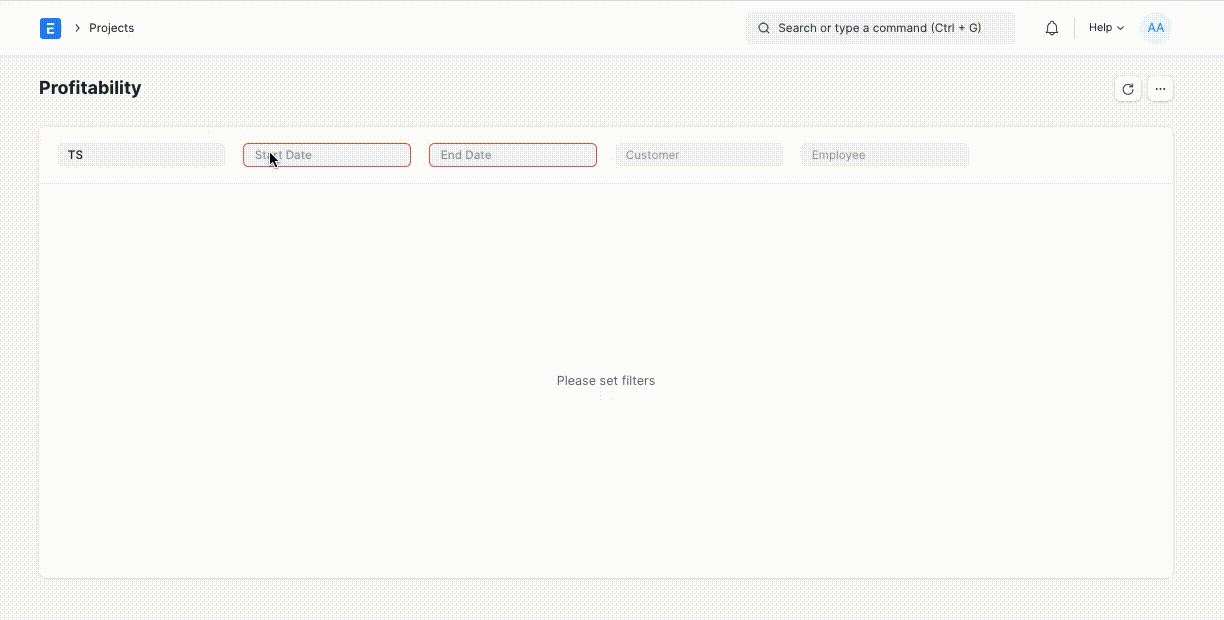# Project Profitability Report

The Project Profitability Report shows the Profitability and Utilization of each employee based on the Timesheets generated.

To view this report, you can go to:

> Home > Projects > Reports > Project Profitability

This report shows the Project Profitability with the following data:

• Timesheet
• Salary Slip generated using the Timesheet
• Sales Invoice generated using the Timesheet### Calculation of Utilization

The Utilization of the Employee is calculated using the Total Billed Hours from the Timesheet, Working Days from the Salary Slip, and the Standard Working Hours from HR Settings.

The formula for Utilization is as follows,

``````Utilization = Total Billed Hours / (Working Days * Standard Working Hours)
``````

### Calculation of Profit

The Profit is calculated using the Gross Pay from the Salary Slip, Grand Total from the Sales Invoice, and Utilization.

The formula for Profit is as follows,

``````Profit = Grand Total - Gross Pay * Utilization
``````

### Calculation of Fractional Cost

The Fractional Cost is calculated using Gross Pay from Salary Slip and Utilization.

The formula for Fractional Cost is as follows,

``````Fractional Cost = Gross Pay * Utilization
``````